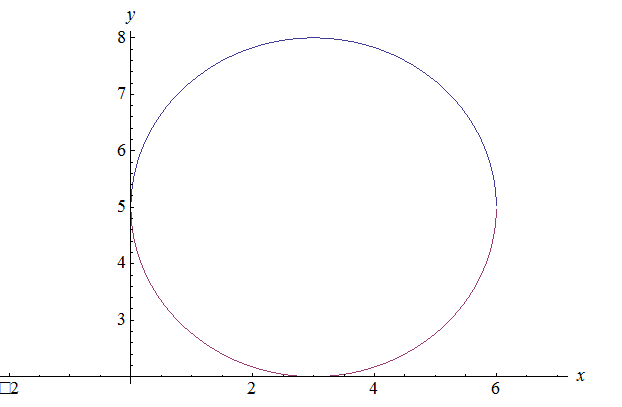# Write an equation of the circle with centre ( h , k ) and radius r .### Precalculus: Mathematics for Calcu...

6th Edition
Stewart + 5 others
Publisher: Cengage Learning
ISBN: 9780840068071### Precalculus: Mathematics for Calcu...

6th Edition
Stewart + 5 others
Publisher: Cengage Learning
ISBN: 9780840068071

#### Solutions

Chapter 1, Problem 26RCC
To determine

## Write an equation of the circle with centre (h,k) and radius r .

Expert Solution

(xh)2+(yk)2=r2

### Explanation of Solution

Given information:

Write an equation of the circle with centre (h,k) and radius r .

Calculation:

The equation for a circle is degree 2 in both variables, having the same coefficient on both sides.

(x3)2+(y5)2=9

Plot of the equation,The graph shows that the centre of the circle is at (3,5) with radius 9=3

On comparing these values to the general equation of circle,

Hence, equation of circle is (xh)2+(yk)2=r2

### Have a homework question?

Subscribe to bartleby learn! Ask subject matter experts 30 homework questions each month. Plus, you’ll have access to millions of step-by-step textbook answers!